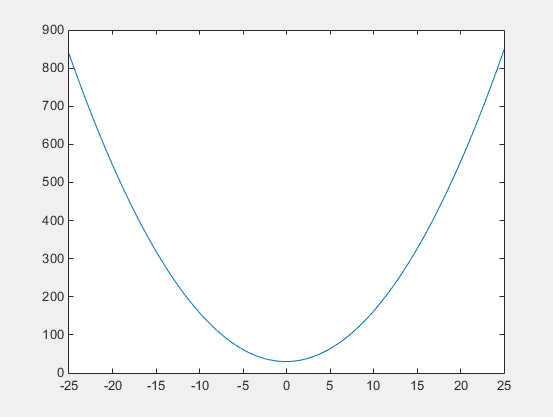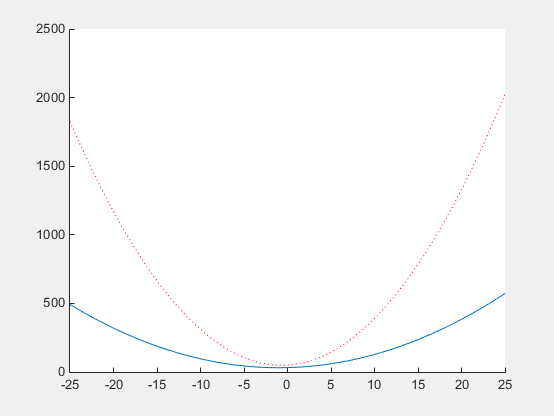## Nested Functions

### What Are Nested Functions?

A nested function is a function that is completely contained within a parent function. Any function in a program file can include a nested function.

For example, this function named `parent` contains a nested function named `nestedfx`:

```function parent disp('This is the parent function') nestedfx function nestedfx disp('This is the nested function') end end```

The primary difference between nested functions and other types of functions is that they can access and modify variables that are defined in their parent functions. As a result:

• Nested functions can use variables that are not explicitly passed as input arguments.

• In a parent function, you can create a handle to a nested function that contains the data necessary to run the nested function.

### Requirements for Nested Functions

• Typically, functions do not require an `end` statement. However, to nest any function in a program file, all functions in that file must use an `end` statement.

• You cannot define a nested function inside any of the MATLAB® program control statements, such as `if/elseif/else`, `switch/case`, `for`, `while`, or `try/catch`.

• You must call a nested function either directly by name (without using `feval`), or using a function handle that you created using the `@` operator (and not `str2func`).

• All of the variables in nested functions or the functions that contain them must be explicitly defined. That is, you cannot call a function or script that assigns values to variables unless those variables already exist in the function workspace. (For more information, see Resolve Error: Attempt to Add Variable to a Static Workspace..)

### Sharing Variables Between Parent and Nested Functions

In general, variables in one function workspace are not available to other functions. However, nested functions can access and modify variables in the workspaces of the functions that contain them.

This means that both a nested function and a function that contains it can modify the same variable without passing that variable as an argument. For example, in each of these functions, `main1` and `main2`, both the main function and the nested function can access variable `x`:

 ```function main1 x = 5; nestfun1 function nestfun1 x = x + 1; end end``` ```function main2 nestfun2 function nestfun2 x = 5; end x = x + 1; end```

When parent functions do not use a given variable, the variable remains local to the nested function. For example, in this function named `main`, the two nested functions have their own versions of `x` that cannot interact with each other:

```function main nestedfun1 nestedfun2 function nestedfun1 x = 1; end function nestedfun2 x = 2; end end```

Functions that return output arguments have variables for the outputs in their workspace. However, parent functions only have variables for the output of nested functions if they explicitly request them. For example, this function `parentfun` does not have variable `y` in its workspace:

```function parentfun x = 5; nestfun; function y = nestfun y = x + 1; end end```

If you modify the code as follows, variable `z` is in the workspace of `parentfun`:

```function parentfun x = 5; z = nestfun; function y = nestfun y = x + 1; end end```

### Using Handles to Store Function Parameters

Nested functions can use variables from three sources:

• Input arguments

• Variables defined within the nested function

• Variables defined in a parent function, also called externally scoped variables

When you create a function handle for a nested function, that handle stores not only the name of the function, but also the values of externally scoped variables.

For example, create a function in a file named `makeParabola.m`. This function accepts several polynomial coefficients, and returns a handle to a nested function that calculates the value of that polynomial.

```function p = makeParabola(a,b,c) p = @parabola; function y = parabola(x) y = a*x.^2 + b*x + c; end end```

The `makeParabola` function returns a handle to the `parabola` function that includes values for coefficients `a`, `b`, and `c`.

At the command line, call the `makeParabola` function with coefficient values of `1.3`, `.2`, and `30`. Use the returned function handle `p` to evaluate the polynomial at a particular point:

```p = makeParabola(1.3,.2,30); X = 25; Y = p(X)```
```Y = 847.5000```

Many MATLAB functions accept function handle inputs to evaluate functions over a range of values. For example, plot the parabolic equation from `-25` to `+25`:

`fplot(p,[-25,25])`You can create multiple handles to the `parabola` function that each use different polynomial coefficients:

```firstp = makeParabola(0.8,1.6,32); secondp = makeParabola(3,4,50); range = [-25,25]; figure hold on fplot(firstp,range) fplot(secondp,range,'r:') hold off```### Visibility of Nested Functions

Every function has a certain scope, that is, a set of other functions to which it is visible. A nested function is available:

• From the level immediately above it. (In the following code, function `A` can call `B` or `D`, but not `C` or `E`.)

• From a function nested at the same level within the same parent function. (Function `B` can call `D`, and `D` can call `B`.)

• From a function at any lower level. (Function `C` can call `B` or `D`, but not `E`.)

```function A(x, y) % Main function B(x,y) D(y) function B(x,y) % Nested in A C(x) D(y) function C(x) % Nested in B D(x) end end function D(x) % Nested in A E(x) function E(x) % Nested in D disp(x) end end end```

The easiest way to extend the scope of a nested function is to create a function handle and return it as an output argument, as shown in Using Handles to Store Function Parameters. Only functions that can call a nested function can create a handle to it.